All the roads of a city are either perpendicular or parallel to one another. The roads are all straight. Roads A, B, C, D and E are parallel to one another. Roads G, H, I, J, K, L and M are parallel to one another.

If road E is between B and C, then distance between A and D is :

###### Option:
A. ½ km
B. 1 km
C. 1.5 km
D. 1.5 - 2 km
E. 2 - 2.5 km
Answer: D . 1.5 - 2 km

Justification:

Clearly from statements (1) and (2), figure 1 follows; from statement (3), figure 2 follows; from statement (4), figure 3 follows; and from statement (5), figure 4 follows; and from statements (6) and (7), figure 5 follows.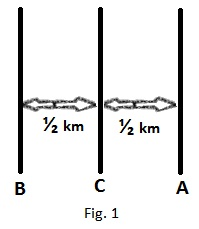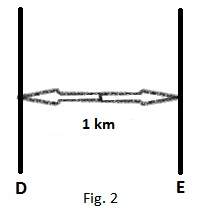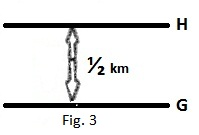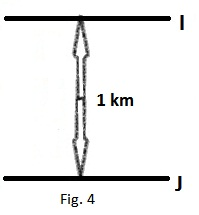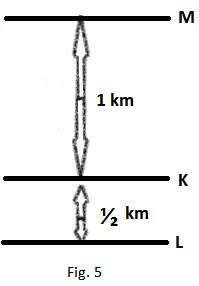From the figure in Q. 50, the distance between A and D
= AB + ED - BE = (1 + 1 - ¼) = 2 - .25  i.e. between 1.5 to 2 km.